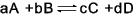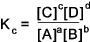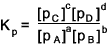Chemistry Index

## Equilibrium

 Equilibrium A chemical reaction is in dynamic equilibrium when the forward reactions and the backward reactions are proceeding at the same rate... Consider the reaction expressed by the stoichiometric equation..A and B are the reactants and C & D are the products.   a ,& b, c and d are the number of moles of each of the substances. It has been found thatThis relationship is known as the equilibrium law or the law of chemical equilibrium.   Where Kc is the equilibrium constant at a given constant... By convention the productions on the right of the reaction equation are the on the top of the equilibrium equation (numerator) and the reactants on the left of the reaction equation are on the bottom line (denominator)or the equilibrium equation The value of Kc provides an indication of the direction of the reaction.   A low value indicates that the [C] [D] are small compared to [A][D] and the backward reaction dominates.  If [A] or/and [B] is increased the reaction would move to the right causing [C][D] to increase to maintain Kc . The relationship as expressed above is based on concentration measurements and a knowledge of molar concentrations in m .dm-3 (m /litre). For reaction involving gases it is more convenient to express the quantities in partial pressures.  In this case the constant is identified as Kp the reaction constant for any gas phase reaction at constant temperature.. For the gaseous state reaction expressed by the stoichiometric equation..The following relationshipThe above equilibrium constants are based on the temperature.  If the forward reaction is exothermic the value of the equilibrium constant decreases as the temperature rises.  If the forward reaction is endothermic the equilibrium constant increases as the temperature rises..(More notes to follow)

 Relevant links.. Chemical Equilibrium... Clear study Notes ChemWeb Online... A great amount of related information< Equilibrium ... Including a page on chemical equilibrium
Chemistry Index